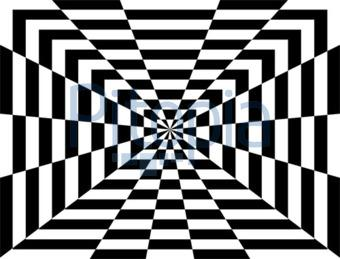#### Howdy, Stranger!

We are about to switch to a new forum software. Until then we have removed the registration on this forum.

# fill complex shapes/rectangles

edited November 2014

Hi :) at first my code:

``````//processing step1
void setup (){

size (600, 600);

// corner_lines
line (300,300,600,0);
line (300,300,600,600);
line (300,300,0,600);
line (300,300,0,0);

// rectangles
float rect_x = 280;
float rect_y = 280;
int rect_width = 40;
int rect_height = 40;
noFill();

for (int i=1; i<9; i++) {
rect (rect_x, rect_y, rect_width, rect_height);
rect_x = rect_x - 35;
rect_y = rect_y - 35;
rect_width = rect_width + 70;
rect_height = rect_height + 70;
}

// lines_left
float endx1 = 0;
float endy1 = random(40,150);

for (int i = 1; i<=4; i++){
line(width/2,height/2,endx1,endy1);
endy1 = endy1 + random(40,150);
}

// lines_right
float endx2 = 600;
float endy2 = random(40,150);

for (int i = 1; i<=4; i++){
line(width/2,height/2,endx2,endy2);
endy2 = endy2 + random(40,150);
}

// lines_top
float endx3 = random(40,150);
float endy3 = 0;

for (int i = 1; i<=4; i++){
line(width/2,height/2,endx3,endy3);
endx3 = endx3 + random(40,150);
}

// lines_buttom
float endx4 = random(40,150);
float endy4 = 600;

for (int i = 1; i<=4; i++){
line(width/2,height/2,endx4,endy4);
endx4 = endx4 + random(40,150);
}

}
``````

I know it could be a better structure ...but I haven´t so many skills until now :-S

The problem is, that I don´t know how to fill out every second shape in my sketch (look at the attachment/picture). Is there a function which can help me?Tagged:

• edited November 2014

Your problem description is very clear. And it is very good to post your code so far. Unfortunately, I don't see any easy way to get from your existing code to the intended visual.

What would help is if you would define your shape in terms of faces made from vertices. Currently you have lines. While these result in the correct shape outline, there are no actual 'computational faces' to color. One could use a color-filling technique (think: paint bucket in photoshop) but that seems a bit convulated.

For this type of image, the most useful method is filter(INVERT). You can draw the rectangles in one PGraphics (i.e. main), then the star burst in another PGraphics (i.e. mask). Then display the main as normal. Then invert the colors of the main, mask it using the star burst PGraphics, then display the masked & inverted image.

You can try this yourself! :-)

Here is an implementation of the described method...

Code Example (not optimized for speed)

``````PGraphics main, mask;

float stepSize       = 50;
int numFans          = 23;
float rotationSpeed  = 0.0035;

void setup() {
size(1280, 720);
main = createGraphics(width, height, JAVA2D);
drawMain();
}

void draw() {
image(main, 0, 0);
}

void drawMain() {
main.beginDraw();
main.rectMode(CENTER);
main.noStroke();
float w = width;
int count = 0;
while (w > 10) {
main.fill(count % 2 == 0 ? 255 : 0);
main.rect(width/2, height/2, w + 7, w);
w -= stepSize;
count++;
}
main.endDraw();
}

float r = width;
float theta = TWO_PI / (numFans-1);
mask.vertex(width/2, height/2); // middle point of the fan
for (int i=0; i<numFans; i++) {
float t = theta * i - frameCount * rotationSpeed;
float x = width/2 + sin(t) * r;
float y = height/2 + cos(t) * r;
mask.fill(i % 2 == 0 ? 255 : 0);
}
}

PImage inverted = main.get(); // 1. get the main image
inverted.filter(INVERT);      // 2. invert the colors
image(inverted, 0, 0);        // 4. display the masked image
}
``````
• I believe I've got it optimized a lil'...: (*)

``````/**
* Inverted Triangle Fans (v2.1)
* by  Amnon (2014/Nov/10)
* mod GoToLoop
*
* forum.processing.org/two/discussion/8031/
* fill-complex-shapesrectangles
*/

PImage bg, inverted;

static final short NUM_FANS = 23, STEP_SIZE = 50;
static final float ROT_SPD  = .0035, THETA = TAU/(NUM_FANS-1);

void setup() {
size(1280, 720, JAVA2D);
noSmooth();
frameRate(60);

inverted = createInverted(bg = createBG());
}

void draw() {
background(bg);
}

PImage createBG() {
pg.smooth(4);
pg.rectMode(CENTER);

for (int i = 0, w = width; w > 10; w -= STEP_SIZE) {
pg.fill((i++&1) == 0? -1 : 0);
pg.rect(width>>1, height>>1, w+7, w);
}

pg.endDraw();
return pg.get();
}

PImage createInverted(PImage img) {
PImage inv = img.get();
inv.filter(INVERT);
return inv;
}

PGraphics pg = createGraphics(width, height, JAVA2D);
pg.beginDraw();

pg.smooth(0);
pg.noStroke();

pg.endDraw();
return pg;
}

float spd = ROT_SPD*frameCount, r = width;
int i = 0, cw = width>>1, ch = height>>1;

m.beginShape(TRIANGLE_FAN);
m.vertex(cw, ch); // middle point of the fan

while (i != NUM_FANS) {
float t = THETA*i - spd;
float x = cw + sin(t)*r, y = ch + cos(t)*r;

m.fill((i++&1) == 0? -1 : 0);
m.vertex(x, y);
}

m.endShape();
m.endDraw();
}

void drawInvertedMasked(PGraphics m, PImage inv) {